### Gaussian 03 Online Manual Last update: 2 July 2006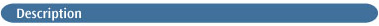This keyword requests a classical trajectory calculation [177,178,179,180] using the Atom Centered Density Matrix Propagation molecular dynamics model [188,189,190]. This method provides equivalent functionality to Born-Oppenheimer molecular dynamics (see the BOMD keyword) at considerably reduced computational cost .

ADMP belongs to the extended Lagrangian approach to molecular dynamics using Gaussian basis function and propagating the density matrix. The best known method of this type is Car-Parrinello (CP) molecular dynamics , in which the Kohn-Sham molecular orbitals, ψi, are chosen as the dynamical variables to represent the electronic degrees of freedom in the system. CP calculations are usually carried out in a plane wave basis (although Gaussian orbitals are sometimes added as an adjunct [394,395,396]). Unlike plane wave CP, it is not necessary to use pseudopotentials on hydrogen or to use Deuterium rather than hydrogen in the dynamics. Fictitious masses for the electronic degrees of freedom are set automatically  and can be small enough that thermostats are not required for good energy conservation.

ADMP can be performed with semi-empirical, HF, and pure and hybrid DFT models (see availability section below for more details). It can be applied to molecules, clusters and periodic systems. PBC calculations use only the Γ point (i.e., no K-integration).

### OPTIONAL INPUT

Although most jobs will not require it, ADMP calculations can accept some optional input:

```[Initial velocity for atom 1: x y z             Optional initial Cartesian velocities
Initial velocity for atom 2: x y z              (ReadVelocity and ReadMWVelocity options)
...
Initial velocity for atom N: x y z
...]                                                             Entire section is repeated NTraj times
[Atom1, Atom2, E0, Len, De, Be              Optional Morse params. for each diatomic product
...]
Terminate subsection with a blank line.```

First, the initial velocity for each atom is read if the ReadVelocity or ReadMWVelocity option is included. Each initial velocity is specified as a Cartesian velocity in atomic units (Bohr/sec) or as a mass-weighed Cartesian velocity (in amu1/2*Bohr/sec), respectively. One complete set of velocities is read for each requested trajectory computation.

Morse parameter data may also be specified for each diatomic product. The Morse parameter data is used to determine the vibrational excitation of diatomic fragments using the EBK quantization rules. It consists of the atomic symbols for the two atoms, the bond length between them (Len, in Angstroms), the energy at that distance (E0 in Hartrees), and the Morse curve parameters De (Hartrees) and Be (Angstroms-1). This input subsection is terminated by a blank line.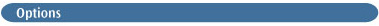MaxPoints=n
Specifies the maximum number of steps that may be taken in each trajectory (the default is 50). If a trajectory job is restarted, the maximum number of steps will default to the number specified in the original calculation.

Lowdin
Use the Löwdin basis for the orthonormal set. The other alternative is Choleski, which uses the Cholesky basis and is the default.

NKE=N
Set the initial nuclear kinetic energy to N microHartrees. NuclearKineticEnergy is a synonym for this option.

DKE=N
Set the initial density kinetic energy to N microHartrees. DensityKineticEnergy is a synonym for this option.

ElectronMass=N
Set the fictitious electron mass to |N/10000| amu (the default is N=1000, resulting in a fictitious mass of 0.1 amu). EMass is a synonym for this option. If N<0, then uniform scaling is used for all basis functions. By default, core functions are weighted more heavily than valence functions.

FullSCF
Do the dynamics with converged SCF results at each point.

Read initial Cartesian velocities from the input stream. Note that the velocities must have the same symmetry orientation as the molecule. This option suppresses the fifth-order anharmonicity correction.

Read initial mass-weighted Cartesian velocities from the input stream. Note that the velocities must have the same symmetry orientation as the molecule. This option suppresses the fifth-order anharmonicity correction.

StepSize=n
Sets the step size in dynamics to n*0.0001 femtoseconds.

BandGap
Whether to diagonalize the Fock matrix in order to report the band gap at each step. The default is NoBandGap.

Restart
Restart an ADMP calculation from the checkpoint file. Note that options set in the original job will continue to be in effect and cannot be modified.

You may also specify alternative isotopes for ADMP jobs using the standard method.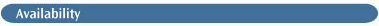Semi-empirical, HF and DFT methods.

The Int=AM1, Int=MNDO or Int=PM3 keyword is required for ADMP jobs using semi-empirical methods. If you require a spin-unrestricted wavefunction, include the UHF keyword as well.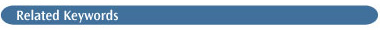BOMD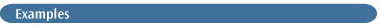The following sample ADMP input file will calculate a trajectory for H2CO dissociating to H2 + CO, starting at the transition state:

```

Dissociation of H2CO --> H2 + CO

0 1
C
O 1 r1
H 1 r2 2 a
H 1 r3 3 b 2 180.

r1 1.15275608
r2 1.74415774
r3 1.09413376
a 114.81897892
b 49.08562961      Final blank line ```

At the beginning of an ADMP calculation, the parameters used for the job are displayed in the output:

```TRJ-TRJ-TRJ-TRJ-TRJ-TRJ-TRJ-TRJ-TRJ-TRJ-TRJ-TRJ-TRJ-TRJ-TRJ-TRJ-TRJ
-------------------------------------------------------------------
INPUT DATA FOR L121
General parameters:
Maximum Steps                =        50
Random Number Generator Seed = 398465
Time Step                    = 0.10000 femptosec
Ficticious electronic mass   = 0.10000 amu
MW individual basis funct.   = True
Initial nuclear kin. energy  = 0.10000 hartree
Initial electr. kin. energy  = 0.00000 hartree
Initial electr. KE scheme  = 0
Multitime step - NDtrC       =    1
Multitime step - NDtrP       =    1
No Thermostats chosen to control nuclear temperature
Integration parameters:

Follow Rxn Path (DVV)       = False
Constraint Scheme           =     12
Projection of angular mom.  = True
Rotate density with nuclei  = True ```

The molecular coordinates and velocities appear at the beginning of each trajectory step (some output digits are truncated here to save space):

```Cartesian coordinates:
I= 1 X= -1.1971360D-01  Y= 0.0000000D+00  Z= -1.0478570D+00
I= 2 X= -1.1971360D-01  Y= 0.0000000D+00  Z=  1.1305362D+00
I= 3 X=  2.8718451D+00  Y= 0.0000000D+00  Z= -2.4313539D+00
I= 4 X=  4.5350603D-01  Y= 0.0000000D+00  Z= -3.0344227D+00
MW Cartesian velocity:
I= 1 X= -4.0368385D+12  Y=  1.4729976D+13 Z=  1.4109897D+14
I= 2 X=  4.4547606D+13  Y= -6.3068948D+12 Z= -2.2951936D+14
I= 3 X= -3.0488505D+13  Y=  6.0922004D+12 Z=  1.8527270D+14
I= 4 X= -1.3305097D+14  Y= -3.1794401D+13 Z=  2.4220839D+14
TRJ-TRJ-TRJ-TRJ-TRJ-TRJ-TRJ-TRJ-TRJ-TRJ-TRJ-TRJ-TRJ-TRJ-TRJ ```

After the trajectory computation is complete, summary information is displayed in the output for each time step in the trajectory:

```Trajectory summary for trajectory     1